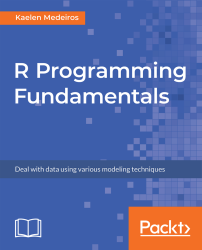•#### R Programming Fundamentals#### Overview of this book

R Programming Fundamentals, focused on R and the R ecosystem, introduces you to the tools for working with data. You’ll start by understanding how to set up R and RStudio, followed by exploring R packages, functions, data structures, control flow, and loops. Once you have grasped the basics, you’ll move on to studying data visualization and graphics. You’ll learn how to build statistical and advanced plots using the powerful ggplot2 library. In addition to this, you’ll discover data management concepts such as factoring, pivoting, aggregating, merging, and dealing with missing values. By the end of this book, you’ll have completed an entire data science project of your own for your portfolio or blog.
PrefaceFree Chapter
Introduction to RData Visualization and GraphicsData ManagementSolutionsOther Books You May Enjoy# Chapter 3: Data Management

The following are the activity solutions for this chapter.

# Activity: Creating and Manipulating Factor Variables

Use the following code to load the datasets library:

`library(datasets)`

Use the following code to load the diamonds dataset:

`data("diamonds")`

The function str() can be used to examine the diamonds dataset as follows:

`str(diamonds)`

We understand that there are three factors of ordered type as we examine the diamonds dataset. To identify the class of those factors, we can use the function class() as follows:

`class(diamonds\$cut)class(diamonds\$color)class(diamonds\$clarity)`

The following code can be used to load the midwest dataset:

`data("midwest")`

The str() function can be...